## It took a crew 9 h 36 min to row 8 km upstream and back again. If the rate of flow of the stream was 2 km/h, what was the rowing speed of th

Question

It took a crew 9 h 36 min to row 8 km upstream and back again. If the rate of flow of the stream was 2 km/h, what was the rowing speed of the crew in still water?

in progress 0
2 weeks 2021-08-29T03:22:18+00:00 1 Answers 0 views 0

3 km/h

Explanation:

Let’s call the rowing speed in still water x, in km/h.

Rowing speed in upstream is: x – 2 km/h

Rowing speed in downstream is: x + 2 km/h

It took a crew 9 h 36 min ( = 9 3/5 = 48/5) to row 8 km upstream and back again. Therefore:

8/(x – 2) + 8/(x + 2) = 48/5      (notice that: time = distance/speed)

Multiplying by x² – 2², which is equivalent to (x-2)*(x+2)

8*(x+2) + 8*(x-2) =  (48/5)*(x² – 4)

Dividing  by 8

(x+2) + (x-2) = (6/5)*(x² – 4)

2*x = (6/5)*x² – 24/5

0 =  (6/5)*x² – 2*x – 24/5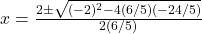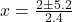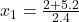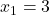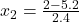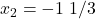A negative result has no sense, therefore the rowing speed in still water was 3 km/h